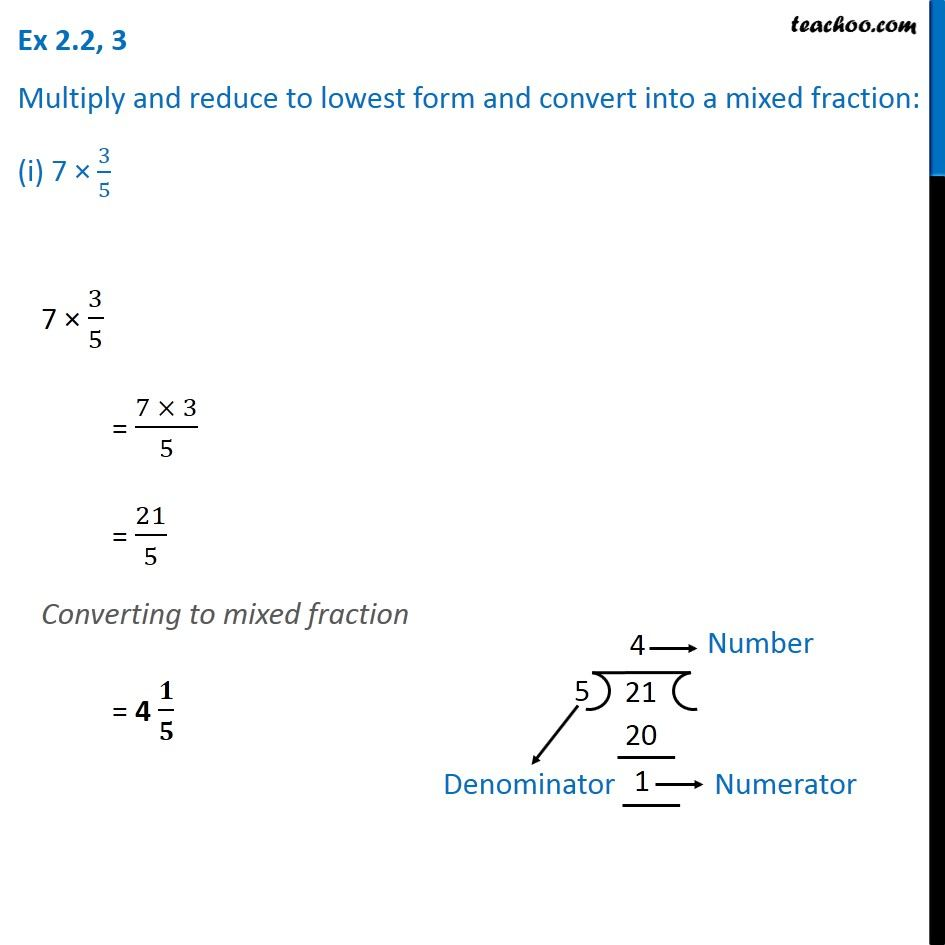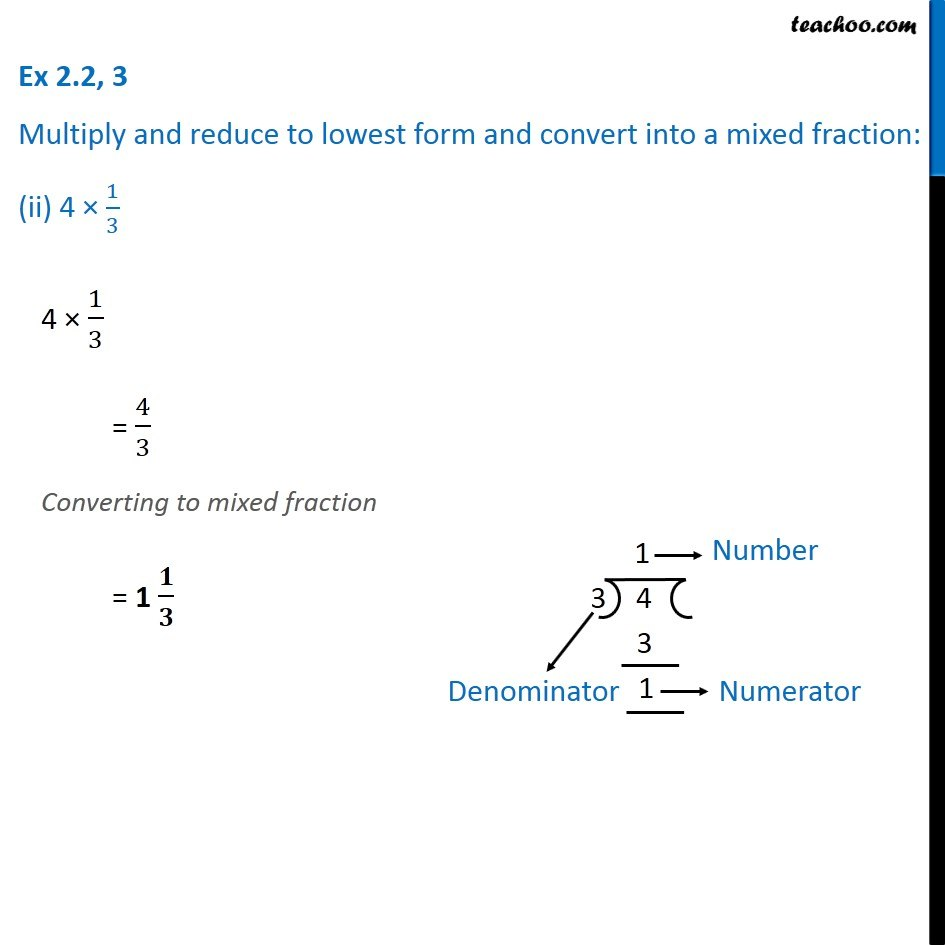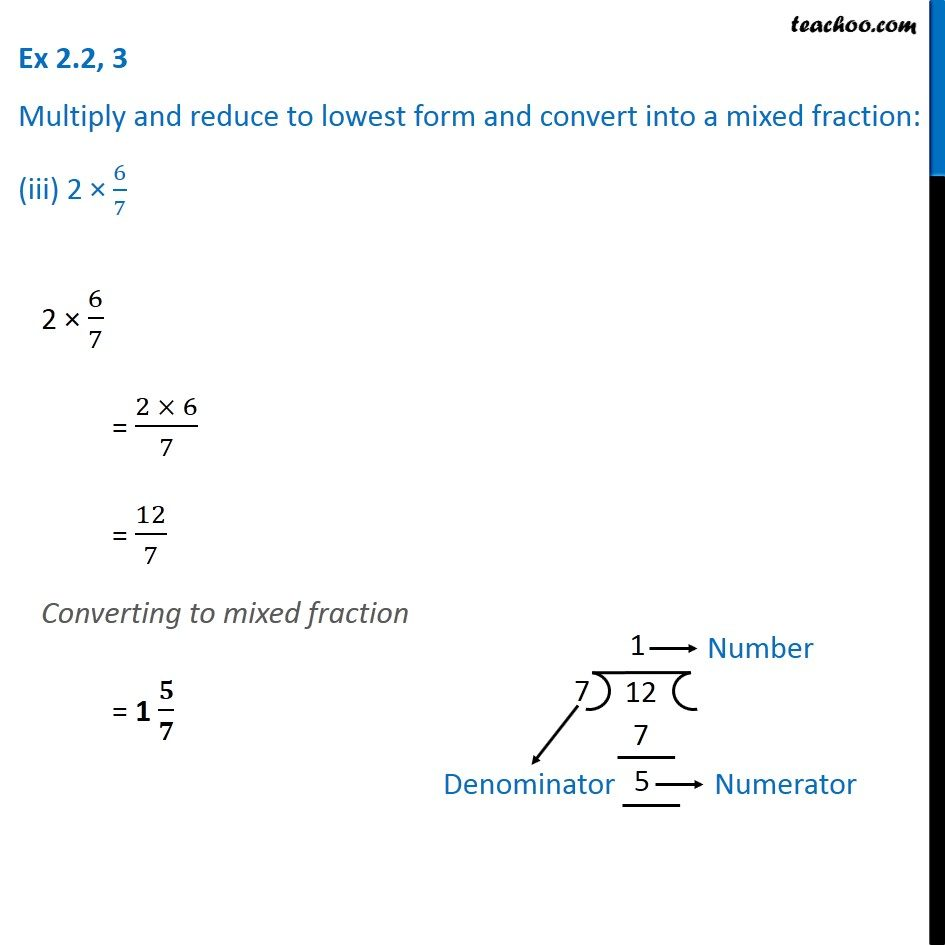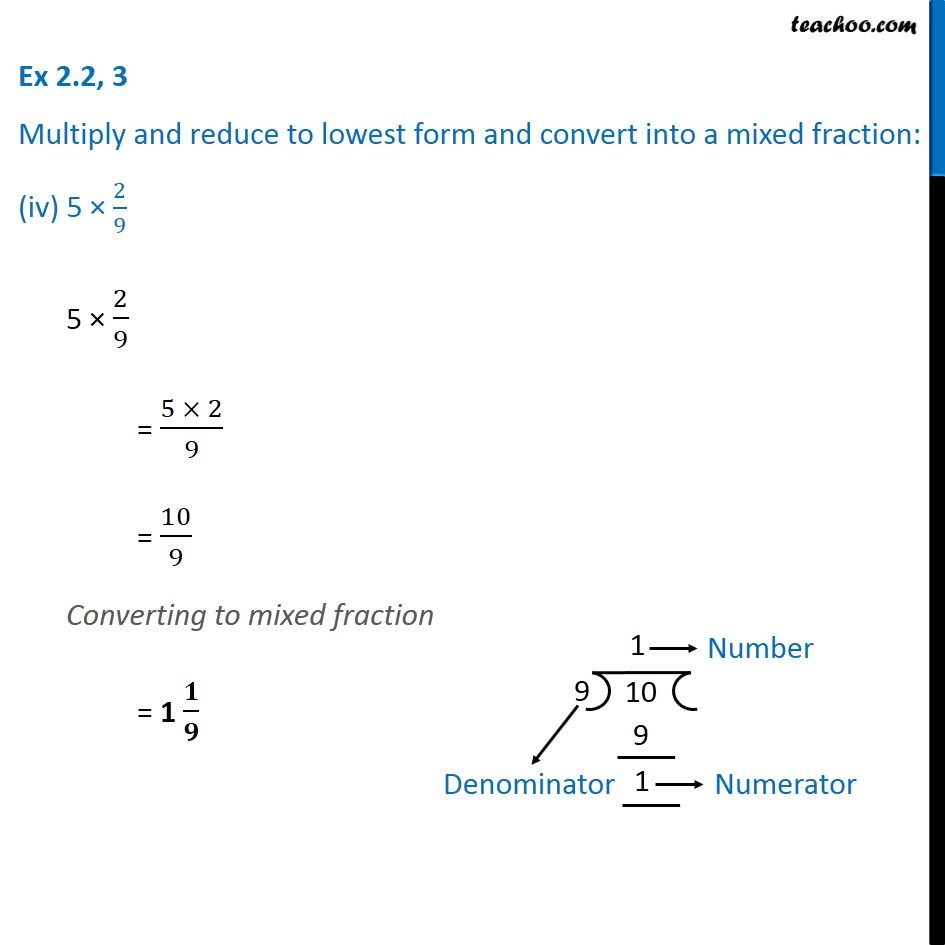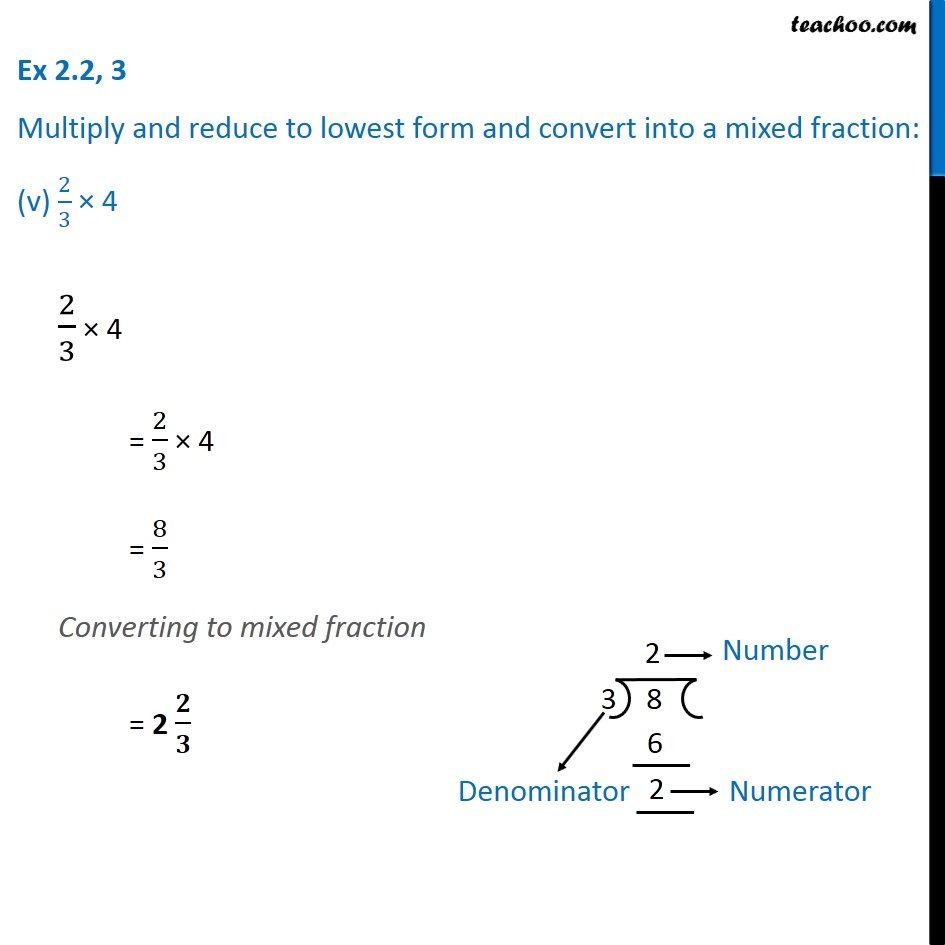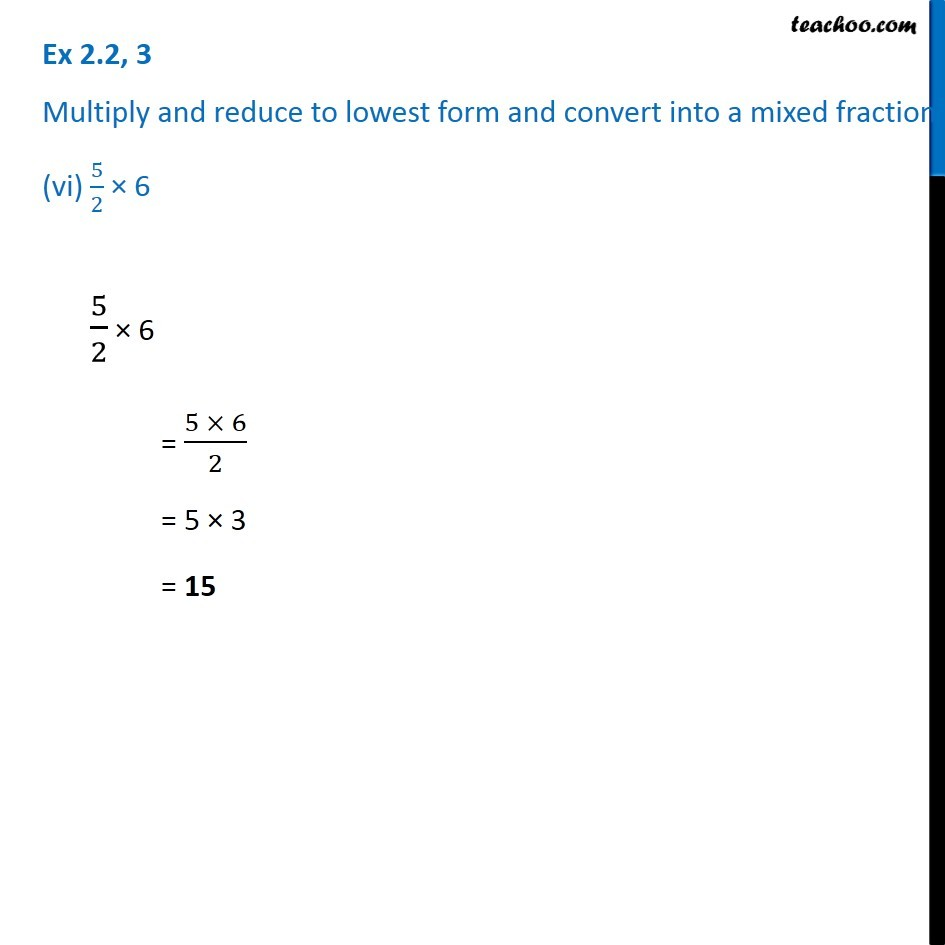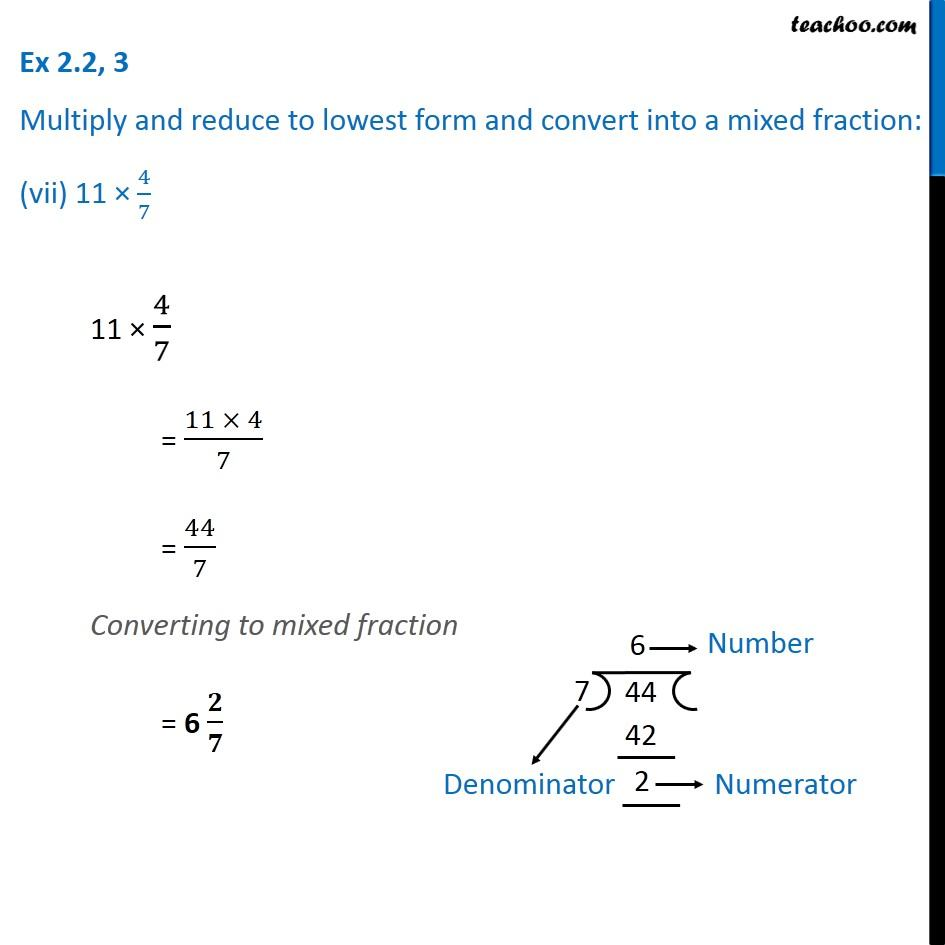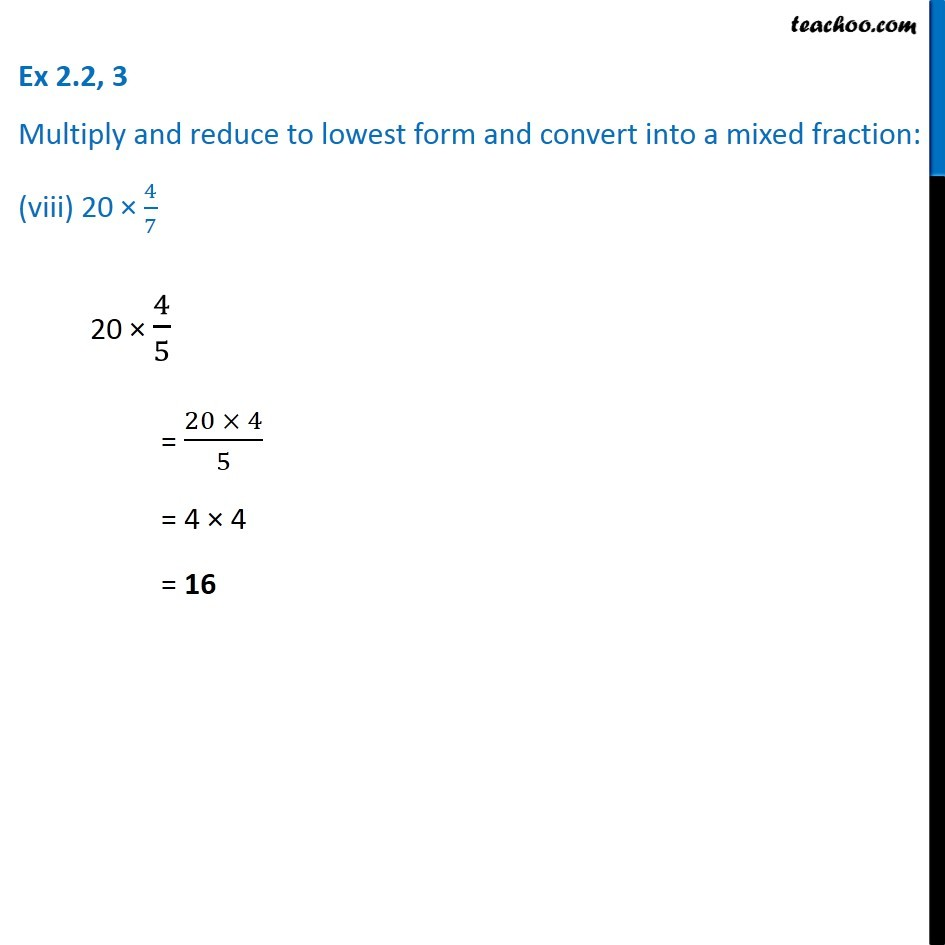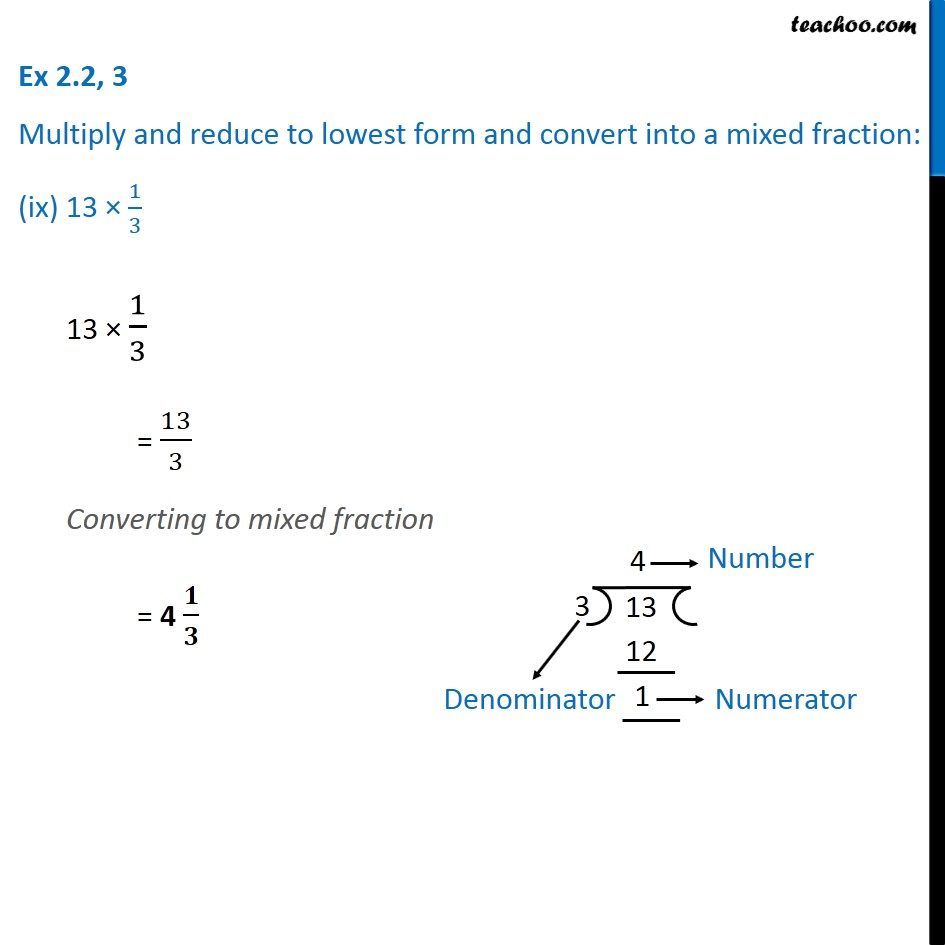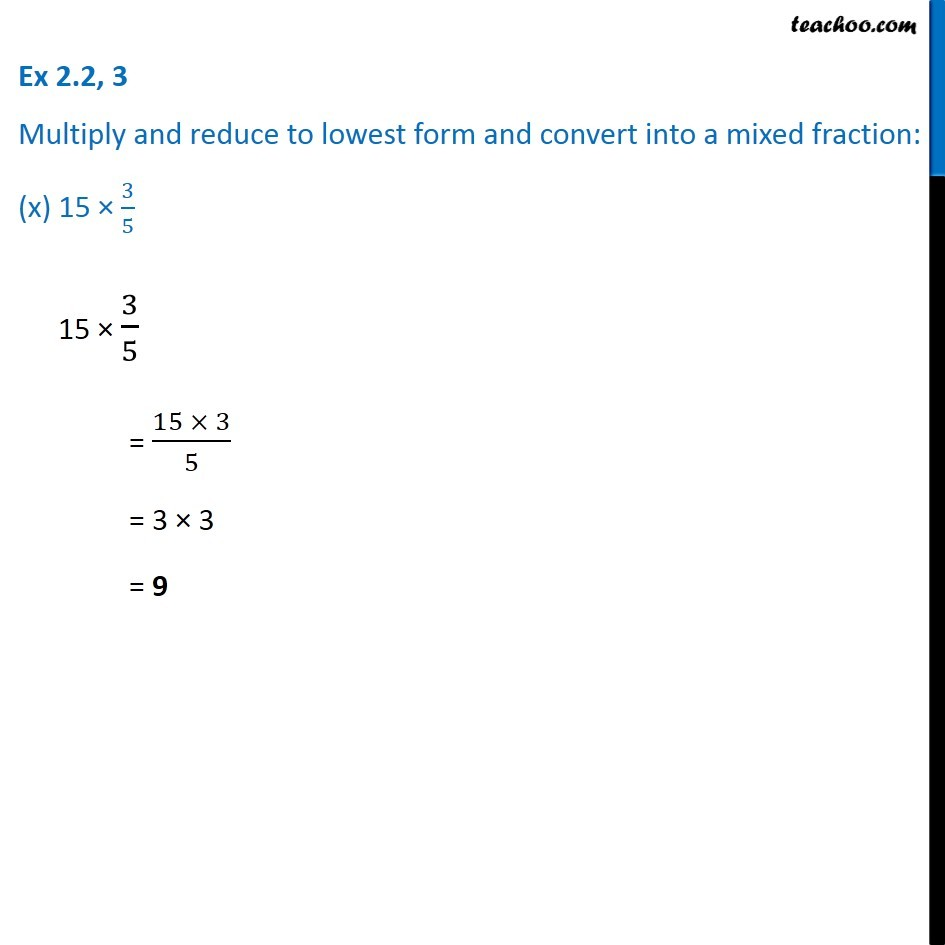Subscribe to our Youtube Channel - https://you.tube/teachoo

1. Chapter 2 Class 7 Fractions and Decimals
2. Serial order wise
3. Ex 2.2

Transcript

Ex 2.2, 3 Multiply and reduce to lowest form and convert into a mixed fraction: (i) 7 × 3/57 × 3/5 = (7 × 3)/5 = 21/5 Converting to mixed fraction = 4 𝟏/𝟓 Ex 2.2, 3 Multiply and reduce to lowest form and convert into a mixed fraction: (ii) 4 × 1/34 × 1/3 = 4/3 Converting to mixed fraction = 1 𝟏/𝟑 2 × 6/7 = (2 × 6)/7 = 12/7 Converting to mixed fraction = 1 𝟓/𝟕 Ex 2.2, 3 Multiply and reduce to lowest form and convert into a mixed fraction: (iv) 5 × 2/95 × 2/9 = (5 × 2)/9 = 10/9 Converting to mixed fraction = 1 𝟏/𝟗 Ex 2.2, 3 Multiply and reduce to lowest form and convert into a mixed fraction: (v) 2/3 × 4 2/3 × 4 = 2/3 × 4 = 8/3 Converting to mixed fraction = 2 𝟐/𝟑 Ex 2.2, 3 Multiply and reduce to lowest form and convert into a mixed fraction: (vi) 5/2 × 65/2 × 6 = (5 × 6)/2 = 5 × 3 = 15 5/2 × 6 = (5 × 6)/2 = 5 × 3 = 15 Ex 2.2, 3 Multiply and reduce to lowest form and convert into a mixed fraction: (vii) 11 × 4/711 × 4/7 = (11 × 4)/7 = 44/7 Converting to mixed fraction = 6 𝟐/𝟕 Ex 2.2, 3 Multiply and reduce to lowest form and convert into a mixed fraction: (viii) 20 × 4/7 20 × 4/5 = (20 × 4)/5 = 4 × 4 = 16 Ex 2.2, 3 Multiply and reduce to lowest form and convert into a mixed fraction: (ix) 13 × 1/313 × 1/3 = 13/3 Converting to mixed fraction = 4 𝟏/𝟑 Ex 2.2, 3 Multiply and reduce to lowest form and convert into a mixed fraction: (x) 15 × 3/5 15 × 3/5 = (15 × 3)/5 = 3 × 3 = 9

Ex 2.2